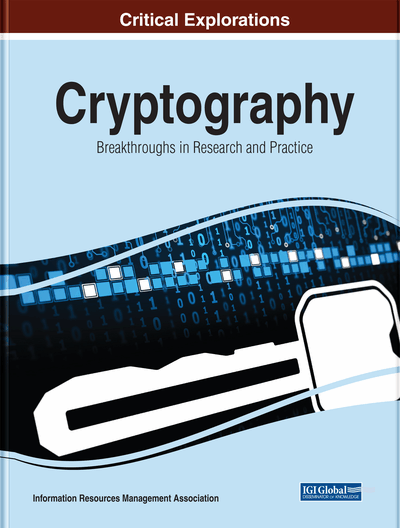# Hybrid Approach of Modified AES

Filali Mohamed Amine (Djellali Liabes University, Algeria) and Gafour Abdelkader (Djellali Liabes University, Algeria)
DOI: 10.4018/978-1-7998-1763-5.ch008

## Abstract

Advanced Encryption Standard is one of the most popular symmetric key encryption algorithms to many works, which have employed to implement modified AES. In this paper, the modification that has been proposed on AES algorithm that has been developed to decrease its time complexity on bulky data and increased security will be included using the image as input data. The modification proposed itself including alteration in the mix column and shift rows transformation of AES encryption algorithm, embedding confusion-diffusion. This work has been implemented on the most recent Xilinx Spartan FPGA.
Chapter Preview
Top

## State Of Art

There are several modified in AES to improve speed the performance, increase the security and addition same complexity on algorithm steps. (Mohammad, et al., 2015) The reason development is appeared many different the implementation on software and hardware. Each implementation has need to modified AES according to the specific proposes.

In (Xu, et al., 2013) a modified AES by used longer key length and data matrix. That extended the data matrix to eight row and variable number of column (6, 8, 12 and 16) the input data block (48, 64, 96 and 128), and extended the key length to (384,512, 768 and 1024). This paper not change the first and fourth stages (substitution byte, Add Round Key), third stage shift row change from shift third row to seventh row shifted left and four stages (mix column) change the static matrix 3x3 to new matrix 8x8, should be calculate inverse static matrix used in Mix column on GF (28). This modification is increase robustness and use a few times for encryption and decryption processing.

In (Kaur, et al., 2014) modified the AES algorithm by reduce the calculation, computation overhead, and reduce the time encryption process. It replaces the mix column stage in AES algorithm into permutation stage (like the permutation table (IP) that used in DES algorithm) because the mix column is take large calculation time and that makes the encryption process are slow. The other stages in AES algorithm don’t change.

Finally, modification used simple S-box for encryption and decryption to reduce the computation amount, the new S-box has some properties, simple generation and same S-box used for encryption and decryption (Rashidi, 2014).

## Complete Chapter List

Search this Book:
Reset